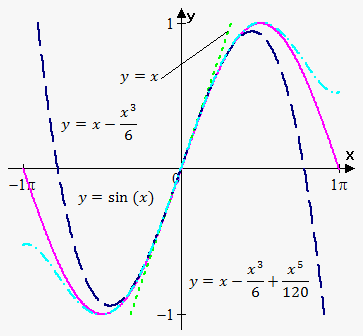# Taylor Polynomial

## Related Calculator: Taylor and Maclaurin (Power) Series Calculator

Suppose that we have n-th degree polynomial p(x)=a_0+a_1(x-a)+a_2(x-a)^2+...+a_(n-1)(x-a)^(n-1)+a_n(x-a)^n, where a,a_0,a_1,a_2,...,a_n are constants.

Now, differentiate this polynomial n times:

p'(x)=a_1+2*a_2(x-a)+3*a_3(x-a)^2+...+(n-1)*a_(n-1)(x-a)^(n-2)+n*a_n(x-a)^(n-1),

p''(x)=1*2*a_2+2*3*a_3(x-a)+...+(n-2)(n-1)a_(n-1)(x-a)^(n-3)+(n-1)na_n(x-a)^(n-2),

p'''(x)=1*2*3*a_3+...+(n-3)(n-2)(n-1)a_(n-1)(x-a)^(n-4)+(n-2)(n-1)na_n(x-a)^(n-3),

..............................................................................................

p^((n))(x)=1*2*3*...*n*a_n.

If we now plug a instead of x in polynomial and all its derivatives we will obtain that

p(a)=a_0, p'(a)=a_1, p''(a)=1*2*a_2, p'''(a)=1*2*3*a_3, ..., p^((n))(a)=1*2*3*...*n*a_n.

Since factorial of number is n! =1*2*3*...*n then

a_0=p(a), a_1=(p'(a))/(1!), a_2=(p''(a))/(2!), ..., a_n=(p^((n))(a))/(n!).

If we now substitute expressions for constants into polynomial we will obtain Taylor Fomula.

Taylor Formula for Polynomials. Polynomialp(x)=p(a)+(p'(a))/(1!)(x-a)+(p''(a))/(2!)(x-a)^2+...+(p^((n-1))(a))/((n-1)!)(x-a)^(n-1)+(p^((n))(a))/(n!)(x-a)^n. is called taylor polynomial at x=a.

With the help of Taylor formula we can write any polynomial in terms of (x-a).

Example 1. Write polynomial p(x)=x^3+3x^2-2x+1 in terms of (x-2).

Here a=2.

We have that p'(x)=3x^2+6x-2, p''(x)=6x+6, p'''(x)=6. All higher-order derivatives will equal 0.

Now, p(2)=2^3+3*2^2-2*3+1=17, p'(2)=3*2^2+6*2-2=22, p''(2)=6*2+6=18, p'''(2)=6.

So,

p(x)=17+(22)/(1!)(x-2)+(18)/(2!)(x-2)^2+(6)/(3!)(x-2)^3=17+22(x-2)+9(x-2)^2+(x-2)^3.

So, equivalently polynomial p(x)=x^3+3x^2-2x+1 can be written as p(x)=(x-2)^3+9(x-2)^2+22(x-2)+17.

Thus, Taylor formula for polynomials allows us to rewrite any polynomial in terms of (x-a).

Now, let's see how we can use this idea for any differentiable functions.

Suppose that function y=f(x) has finite derivatives up to n-th order at point a.

Taylor Formula for any Function. For function y=f(x) n-th degree Taylor polynomial at point x=a is  T_n(x)=f(a)+(f'(a))/(1!)(x-a)+(f''(a))/(2!)(x-a)^2+...+(f^((n-1))(a))/((n-1)!)(x-a)^(n-1)+(f^((n))(a))/(n!)(x-a)^n.

Of course, T_n(x)!=f(x), but as appeared T_n(x) is a very good approximation for f(x) when x->a. And the higher n (order of polynomial) the better approximation.

Fact. T_n(x)~~f(x) as x->a.

This fact allows us to approximate function by polynomial near point x=a with any precision we want, by taking high degree polynomial.

Example 2. Find first, third and fifth degree polynomials for function f(x)=sin(x) nearx=0.

Here a=0.

To find fifth degree polynomial we need derivatives up to fifth order.

We have that f'(x)=cos(x), f''(x)=-sin(x), f'''(x)=-cos(x), f^((4))(x)=sin(x), f^((5))(x)=cos(x).

Now, f(0)=0, f'(0)=1, f''(0)=0, f'''(0)=-1, f^((4))(0)=0, f^((5))(0)=1.

First degree taylor polynomial is T_1(x)=f(0)+(f'(0))/(1!)(x-0)=0+1/1 x=x.

Third degree taylor polynomial is T_3(x)=f(0)+(f'(0))/(1!)(x-0)+(f''(0))/(2!)(x-0)^2+(f'''(0))/(3!)(x-0)^3=0+1/1 x+0/(2) x^2+(-1)/(6)x^3=x-(x^3)/6.

Fifth degree taylor polynomial is T_5(x)=f(0)+(f'(0))/(1!)(x-0)+(f''(0))/(2!)(x-0)^2+(f'''(0))/(3!)(x-0)^3+(f^((4))(0))/(4!)(x-0)^4+(f^((5))(0))/(5!)(x-0)^5=

=0+1/1 x+0/(2) x^2+(-1)/(6)x^3+0/(4!)x^4+1/(120)x^5=x-(x^3)/6+(x^5)/(120).

So,

T_1(x)=x, T_3(x)=x-(x^3)/6, T_5(x)=x-(x^3)/6+(x^5)/120.

Remember, the higher degree of polynomial, the better precision. Figure illustrates this. Note, that approximation good only near point of expansion, in this case a=0.